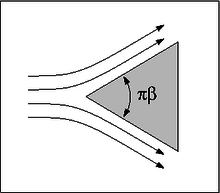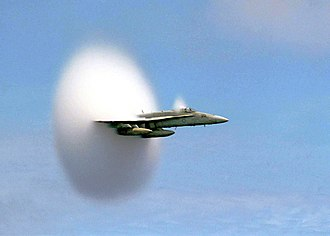# Preface

This section discusses a simplified version of the Adomian decomposition method first concept of which was proposed by Randolf Rach in 1989 that was crystallized later in a paper published with his colleagues G. Adomian and R.E. Meyers. That is way this technique is frequently referred to as the Rach--Adomian--Meyers modified decomposition method (MDM for short). Initially, this method was applied to power series expansions, which was based on the nonlinear transformation of series by the Adomian--Rach Theorem. Similar to the Runge--Kutta methods, the MDM can be implemented in numerical integration of differential equations by one-step methods. In case of polynomials or power series, it shows the advantage in speed and accuracy of calculations when at each step the Adomian decomposition method allows one to perform explicit evaluations.

# Falkner--Skan layer

The Falkner–Skan boundary layer (named after V. M. Falkner and Sylvia W. Skan) describes the steady two-dimensional laminar boundary layer that forms on a wedge, i.e. flows in which the plate is not parallel to the flow. It is a generalization of the Blasius boundary layer. The Prandtl's boundary layer equations for steady incompressible flow with constant viscosity and density can be reduced to the Falkner–Skan equation for single function f(η)

$f''' + f\,f'' + \beta \left[ 1 - \left( f' \right)^2 \right] = 0$
subject to the boundary conditions
$f(0) = f' (0) =0 , \qquad f' (\infty ) = 1.$
Here η is the similarity variable. The Falkner–Skan equation is a generalization of the Blasius equation, with β = 0.Wedge flow An F/A-18 Hornet creating a vapor cone at transonic speed just before reaching the speed of sound

Example: The problem is to find the potential V(x) that satisfies the following second order ordinary differential equation and boundary conditions

$\begin{split} V'' - \left( V' \right)^2 - \frac{x}{2}\,V' + 3\,V &=0 , \\ V' (0) &= 0, \\ V'' (\infty ) &=1. \end{split}$
The connection parameter parameter associated to that problem is:
$V(0) = \gamma \approx$
It is convenient to work with the derivative V'(x) and to perform the following change of variable:
$\begin{split} f(x) &= V' (x) , \\ f(x) &= x\, g(x^2 ) , \\ z &= x^2 , \end{split}$
then the given problem is equivalent to:
$\begin{split} 4z\, g'' - 4z\,g\,g' - 2g^2 + (6-z)\, g' + 2g &=0 , \\ g(+\infty ) &= 1, \end{split}$
with the new connection parameter defined as
$k = g(0) = -3\gamma \approx −0.228598202437021924373656107397047188424054430086 .$
■

Example: The Thomas--Fermi problem for the neutral atom [17,18] writes in terms of a function u(x):

$u'' = \sqrt{\frac{u^3}{x}} ,$
subject to
$u(0) =1, \qquad u(+\infty ) =0 .$
The connection parameter is $$k = u' (0) \approx .$$ With transformation
$g(z) = \sqrt{u(z^2 )} , \qquad z= \sqrt{x} ,$
the original problem is then equivalent to
$\begin{split} z \left[ g\,g'' + \left( g' \right)^2 \right] - g\, g' - 2 z^2 g^3 =0 , \\ g(0) =1 , \\ g(+\infty ) =0 . \end{split}$
The connection parameter becomes
$k = g'' (0) \approx −1.58807102261137532 .$
■

Example: Consider the equation of the flow of a third grade fluid in a porous half space which has been investigated by Hayat et al and Ahmad. The formulation of this problem in terms of f(z) is

$\begin{split} f'' + b_1 \left( f' \right)^2 f'' - \frac{b_1 c}{3}\,f\left( f' \right)^2 - c\,f &=0, \\ f(0) &= 1, \\ f(+\infty ) &= 0, \end{split}$
in which b1 = 0.6 and c = 0.5 are two constants that are assigned the positive numerical values. Using a rescaling of the form
$f(z) = \frac{1}{\sqrt{b_1 c}} \,g\left( z \sqrt{c} \right)$
the problem (39–41) may be written as follows:
$\begin{split} g'' + \left( g' \right)^2 g'' - \frac{1}{3}\,g \left( g' \right)^2 - g &=0, \\ g(0) &= A = \sqrt{b_1 c} , \\ g(+\infty ) &= 0. \end{split}$
The connection parameter is again:
$k = g' (0) \approx −0.678301, \qquad \mbox{for b_1 = 0.6 and c = 0.5}.$
In our conventions, it corresponds to
$\begin{split} A &= \sqrt{b_1 c} \approx 0.5477225575, \\ k &= \sqrt{b_1}\, f' (0) \approx −0.525410 . \end{split}$
■

1. Falkner–Skan boundary layer
2. Falkner V. M. and Skan, S. W. Advisory Committee for Aeronautics, 1930, no 1314, 1930.
3. Falkner V. M. and Skan, S. W. Some approximate solutions of the boundary-layer equations. Philosophical Magazine, 1931, Vol. 12 12 , 865-896 (1931).
4. Abbasbandy, S. and Dervillie, C., analytic continuation of Taylor series and the boundary value problems of some nonlinear ordinary differential equations, 2011,
5. F. Ahmad, A simple analytical solution for the steady flow of a third grade fluid in a porous half space, Communications in Nonlinear Science and Numerical Simulation, 2009, Vol. 14, pp. 2848.
6. Asaithambi, A. A series solution of the Falkner–Skan equation using the crocco–wang transformation, International Journal of Modern Physics, 2017, Vol. 28, No. 11, 1750139; https://doi.org/10.1142/S012918311750139X
7. Fermi, E., Un Metodo Statistico per la Determinazione dialcune Priopriet`a dell’Atomo, Rendiconti Academia Dei Lincei, 1927, Vol. 6, pp. 602--607.
8. T. Hayat, F. Shahzad and M. Ayub, Analytical solution for the steady flow of the third grade fluid in a porous half space, Applied Mathematical Modelling, 2007, Volume 31, Issue 11, November 2007, Pages 2424-2432; https://doi.org/10.1016/j.apm.2006.09.008
9. Kazem, S., Rad, J.A., Parand, K., Abbasbandy, S., A new method for solving steady flow of a third-grade fluid in a porous half space based on radial basis functions, Verlag der Zeitschrift f ̈ur Naturforschung, Tbingen, 2011; doi: 10.5560/ZNA.2011-0014
10. Polchinski, J., Renormalization and effective lagrangians, Nuclear Physics B 1984, Vol. 231 (1984) 269--295.
11. L. H. Thomas, The calculation of atomic fields, Mathematical Proceedings of the Cambridge Philosophical Society, 1927, Vol 23, pp. 542--548. doi:10.1017/S0305004100011683
12. H. Weyl, Concerning the Differential Equations of Some Boundary Layer Problems, Proceedings of the National Academy of Sciience of the United States of America, 1941, Vol. 27, No 12, pp. 578--583; doi: 10.1073/pnas.27.12.578